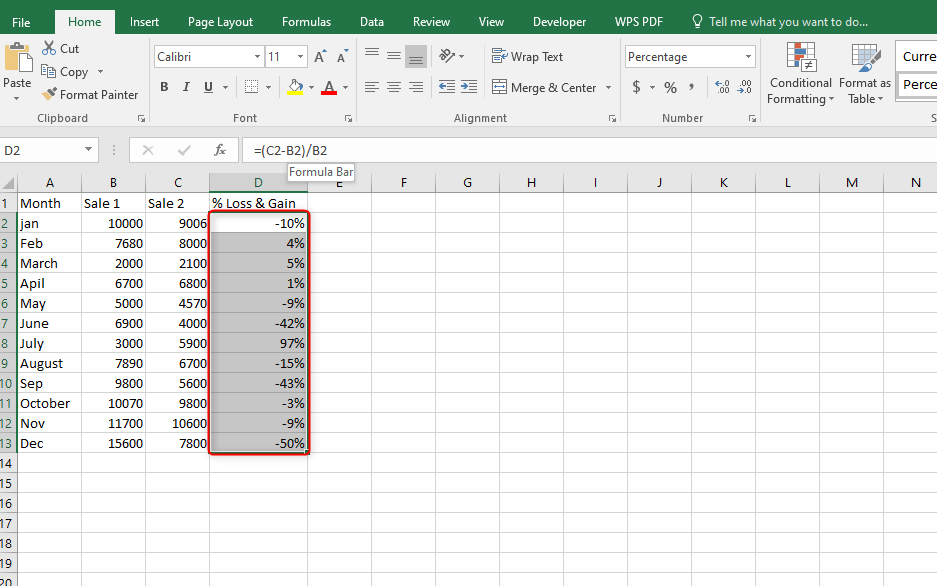# Excel formula for percentage gain or loss

A gain is usually an increase from the previously recorded value. The ability to manipulate Excel's Arithmetic Power is one of the greatest skills needed to calculate the gain or loss. We can, therefore, calculate %gain using the following general mathematics formula:

%gain or loss= (Gain or loss/previous value) *100. To find gain all we need to do is: Subtract the previous value from the latter one. If we have these values supplied, we can easily calculate the percentage gain or loss in Excel.

## Procedure:

1. Have the totals (previous and later values) which will be used.

2. Add a column for % gain or %loss.3. Perform Subtraction on the cells from both the initial and recent values.

Type in the following formula:

=(C2-B2)

This formula alone will be responsible for calculating the gain or loss. Complete the formula so that it becomes similar to:

=(C2-B2)/B24. Press the Percentage symbol to convert the decimals to actual percentages.5. From the results of the calculation, a negative percentage will imply a percentage loss while a positive percentage will indicate again.## Excel formula for percentage change between two percentages

To find the percentage change between two percentages we use the following formula:

=Percentage change/ (first percentage+ second percentage)/2

The percentage change is usually the difference returned from the subtraction of the two percentages: I.e. Percent one-percentage two. We then find the averages of the two percentages to find the dividend to be used in the calculation of the % change of the two percentages. The results are then multiplied by 100.

## Procedure

1. Launch Excel and navigate to your worksheet.

2. Identify the column or cells with the two percentages.3. Outside those two cells insert the following formula:

=(B2-C2)/(B2+C2)/24. Press Enter.

5. Save changes.Excel formula for percentage change for a year over year

To find the percentage change year over year:

= (This year's value-last year's value)/last year's value*100

## Procedure in Excel

1. Launch Excel. Navigate to your worksheet.

2. Add a column for percentage Change year over year.

3. Add a zero at the topmost cell of the column since it coincides with the Beginning year.

4. Type the following formula.

=(B3-B2)/B25. Press enter.6. Highlight the column with decimals and press the percentage symbol under the Number group.7. Drag from the second cell of the column to find the percentage change year over year.